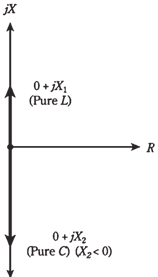## Complex impedances in series Assignment Help

Assignment Help: >> Electrical Engineering - Complex impedances in series

Complex impedances in series:

When there are resistors, coils, and capacitors in series, you must envision the RX plane.

Each component, whether it is an inductor, a resistor, or a capacitor, has an impedance which can be represented as a vector in RX plane. The vectors for resistors are constant regardless the variation in frequency. But vectors for coils and capacitors vary with the frequency, as we have learned.

Pure reactances

The Pure inductive reactances (XL) and capacitive reactances (XC) simply add together when coils and capacitors are in series. Hence, XL + XC. In the RX plane, the vectors add, but because these vectors point in exactly opposite directions inductive reactance upwards and capacitive reactance downwards the resultant sum vector will also inevitably point either straight up or down.Figure --  Pure inductance and pure capacitance are represented by reactance vectors which point straight up and down.

Problem :1

A coil and capacitor are connected in series, with jXL = j200 and jXC = -j150. What is net reactance vector jX?

Just add values jX = jXL + jXC + j200 + (-j150) =  j(200-150) =j50. This is an inductive reactance, as it is positive imaginary.